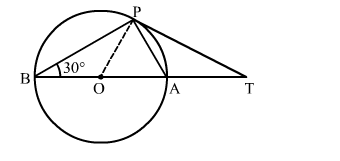# In the given figure, O is the centre of a circle, BOA is its diameter and the tangent at the point`
Question:

In the given figure, O is the centre of a circle, BOA is its diameter and the tangent at the point P meets BA extended at T. If ∠PBO = 30 then ∠PTA = ?
(a) 60
(b) 30
(c) 15
(d) 45Solution:

We know that a chord passing through the centre is the diameter of the circle.
∵∠BPA = 90    (Angle in a semi circle is 90)
By using alternate segment theorem
We have ∠APT = ∠ABP = 30
Now, In △ABP
∠PBA + ∠BPA + ∠BAP = 1800            [Angle sum property of a triangle]
⇒ 30 +  900 + ∠BAP = 180
⇒ ∠BAP = 60
Now, ∠BAP = ∠APT + ∠PTA
⇒ 60 = 30 +  ∠PTA
⇒ ∠PTA = 30
Hence, the correct answer is option (b).